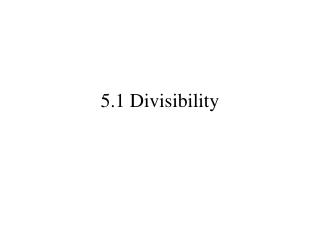DownloadDownload Presentation5.1 Divisibility

# 5.1 Divisibility

Download Presentation## 5.1 Divisibility

- - - - - - - - - - - - - - - - - - - - - - - - - - - E N D - - - - - - - - - - - - - - - - - - - - - - - - - - -
##### Presentation Transcript

1. 5.1 Divisibility

2. Natural Numbers • The set of natural numbers or counting numbers is {1,2,3,4,5,6,…}

3. Factors • The factors of a number are numbers that are multiplied together to equal that number. • Example: What are the factors of 12? So the factors of 12 are 1, 2, 3, 4, 6, & 12. If 12 is divided by any of its factors the remainder is zero.

4. Divisibility • We say a is divisible by b if dividing a by b leaves a remainder of 0. • We say that b is a divisor of a. • Example: Since with no remainder we say that 24 is divisible by 8 8 divides 24 8 is a divisor of 24 We write 8|24

5. Factors and divisibility • Factors and divisors are the same. • For example: 8 is a factoranddivisor of 16 since

6. Review the rules of divisibility p. 144

7. Examples • 4,681,396 is divisible by 2 since 6 is even • 5,931,471 is divisible by 3 since 5 + 9 + 3 + 1 + 4 + 7 + 1 = 30 is divisible by 3 • 4,865,924 is divisible by 4 since 4 | 24 • 954 is divisible by 6 since 2 | 954 and 3 | 954 • 30,385 is divisible by 5 since it ends in 5 or 0 • 593,777,832 is divisible by 8 since the 8|832 • 543,186 is divisible by 9 since 5 + 4 + 3 + 1 + 8 + 6= 27 is divisible by 9 • 35,780 is divisible by 10 since it ends in 0 • 614,608,176 is divisible by 12 since 3 and 4 divide it

8. Prime Numbers • A prime number is a number greater than 1 with only 2 divisors or factors; 1 and itself. • Example: 2, 3, 5, 7, 11, 13, 17, … • Activity: Sieve of Eratosthenes

9. Composite Numbers • A composite number is a number > 1 with a factor other than 1 and itself. • For example: 4, 6, 8, 9, 10, 12, 14, 15,…

10. Prime Factorization • The prime factorization of a number is expressing it as a product of its prime factors.

11. Factor Trees • We can show prime factorization using a factor tree: 340 34 10 2 17 2 5 So 340 =

12. Write a factor tree for the following numbers • 700 • 180 • 510

13. Greatest Common Factor • The Greatest Common Factor or GCF is the greatest divisor of all the numbers. • To find: • Write the prime factorization of each number • Select factors that are common to each • Take the smallest power of each of the factors selected • Multiply

14. Examples • Find the GCF of 225 and 825 • Find the GCF of 72 and 120

15. Relatively Prime • If two numbers share no common factors other than one then they are called relatively prime. • Example: 35 and 12 are relatively prime since they share no common factors other than 1

16. Least Common Multiple • The Least Common Multiple is the smallest number divisible by all of the numbers. • One way to find the LCM is to list all multiples of each number and circle the smallest common one • Example: To find the LCM of 15 and 20 Multiples of 15: 15, 30, 45, 60, 75,… Multiples of 20: 20, 40, 60, 80,… The LCM of 15 and 20 is 60.

17. 2nd Way to Find LCM • Write prime factorization of each number • Select every factor • Take the highest power of each factor • Multiply

18. Example • Find the LCM of 18 and 30 • Find the LCM of 144 and 300 • Find the LCM of 60 and 108

19. HW: p. 200/1-10,25-68